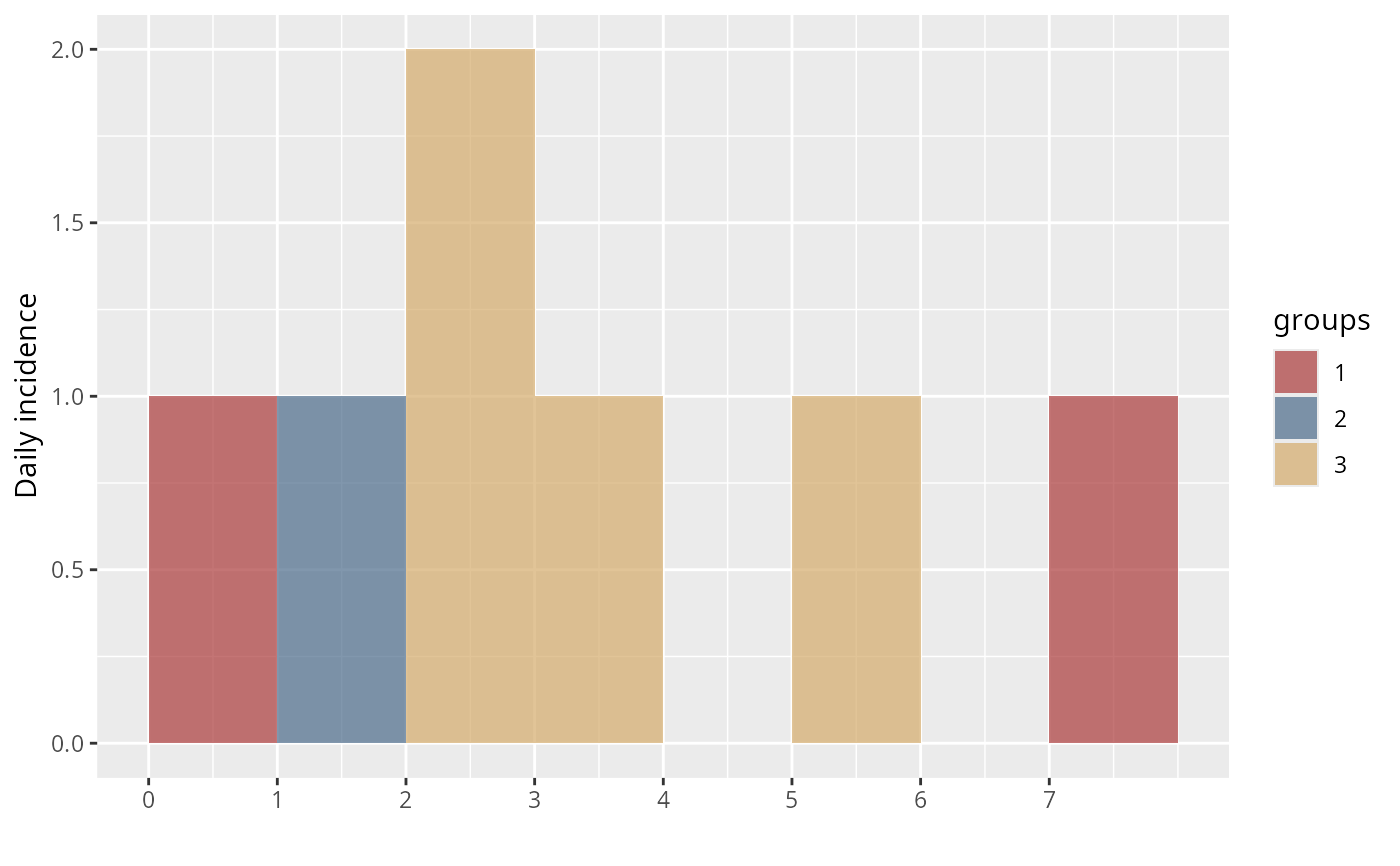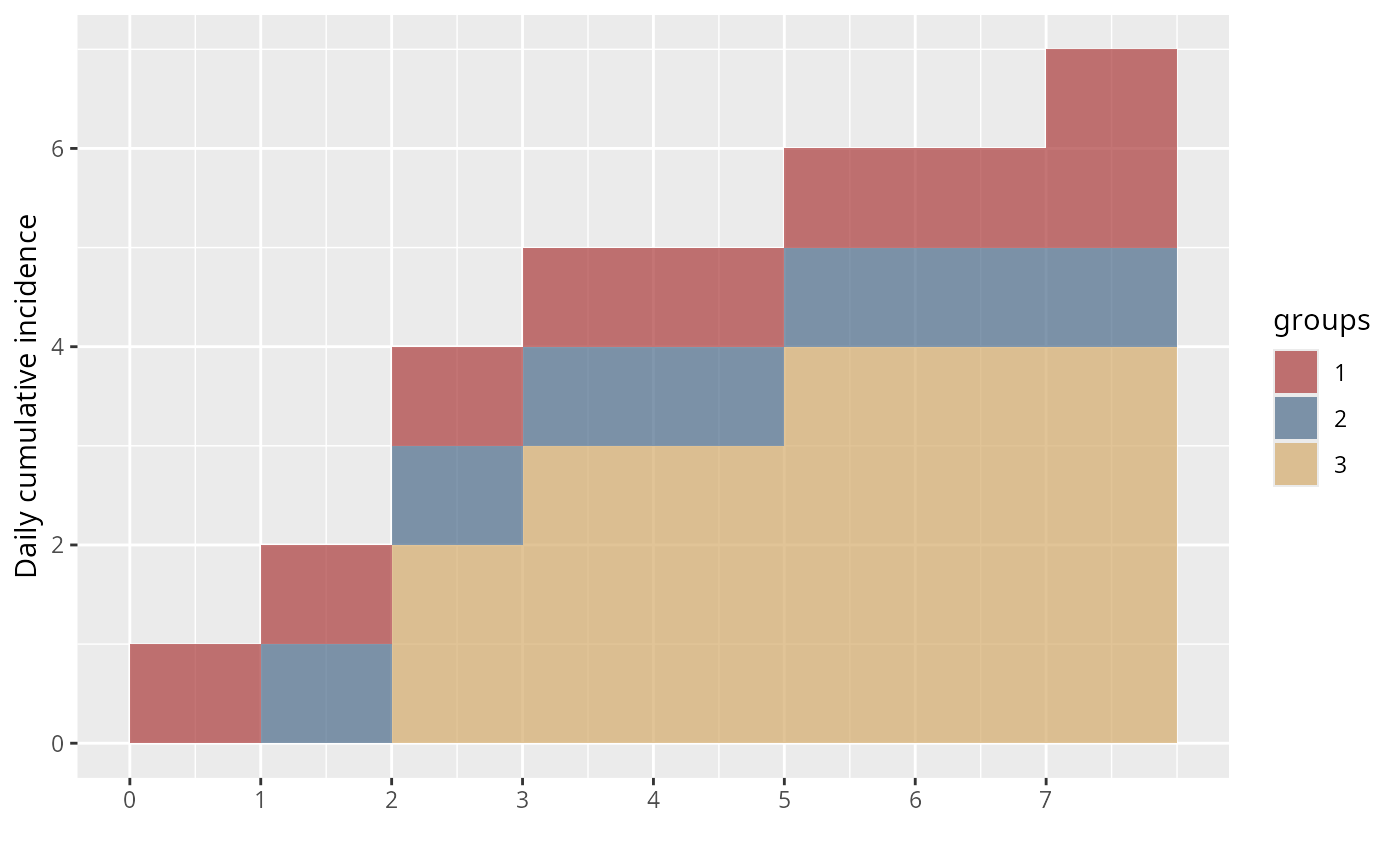cumulate is an S3 generic to compute cumulative numbers, with methods for different types of objects:

cumulate(x)

# S3 method for default
cumulate(x)

# S3 method for incidence
cumulate(x)

## Arguments

x An incidence object.

## Details

• default method is a wrapper for cumsum

• incidence objects: computes cumulative incidence over time

• projections objects: same, for projections objects, implemented in the similarly named package; see ?cumulate.projections for more information, after loading the package

The incidence() function to generate the 'incidence' objects.

## Examples

dat <- as.integer(c(0,1,2,2,3,5,7))
group <- factor(c(1, 2, 3, 3, 3, 3, 1))
i <- incidence(dat, groups = group)
i#> <incidence object>
#> [7 cases from days 0 to 7]
#> [3 groups: 1, 2, 3]
#>
#> $counts: matrix with 8 rows and 3 columns #>$n: 7 cases in total
#> $dates: 8 dates marking the left-side of bins #>$interval: 1 day
#> $timespan: 8 days #>$cumulative: FALSE
#> plot(i)i_cum <- cumulate(i)
i_cum#> <incidence object>
#> [7 cases from days 0 to 7]
#> [3 groups: 1, 2, 3]
#>
#> $counts: matrix with 8 rows and 3 columns #>$n: 7 cases in total
#> $dates: 8 dates marking the left-side of bins #>$interval: 1 day
#> $timespan: 8 days #>$cumulative: TRUE
#> plot(i_cum)